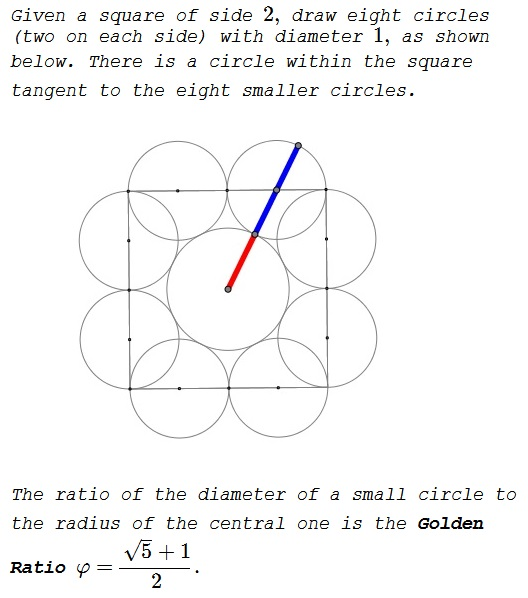# Golden Ratio in Square And Circles

The Golden Ratio pops up in a modification of problem 22 from the 2014 American Mathematical Competition.The claim will become obvious from the solution to the AMC's problem:

Eight semicircles line the inside of a square with side length of $2$ as shown.What is the radius of the circle tangent to all of these semicircles?

(A)  $\displaystyle \frac{1+\sqrt{2}}{4}$  (B)  $\displaystyle \frac{\sqrt{5}-1}{2}$  (C)  $\displaystyle \frac{1+\sqrt{3}}{4}$
(D)  $\displaystyle \frac{2\sqrt{3}}{5}$  (E)  $\displaystyle \frac{\sqrt{5}}{3}$

The answer is obvious from the diagram below: the right triangle has sides $2$ and $1$ and, therefore the hypotenuse of length $\sqrt{5}.$One half the of the hypotenuse $\displaystyle\left(\frac{\sqrt{5}}{2}\right)$ is the sum of the radii of the central circle and the small ones which is $\displaystyle \frac{1}{2}.$ Thus we deduce that the radius of the central circle is $\displaystyle \frac{\sqrt{5}}{2}-\frac{1}{2}=\frac{\sqrt{5}-1}{2}.$

This leads to the Golden Ratio, as above.

### Golden Ratio